#### For Calculated questions, you can use wildcards (eg. {x} , {y}) when taking the quiz to create numeric questions that are replaced with randomly generated numbers. For example, if you want to create a question with many “rectangular calculation areas,” create a question with two wildcards (eg. {base}, {height}) and so can enter {base}*{height} in the input field of “Correct formula=.”

Refer to:
10-01-20.How to use quiz sample questions in your course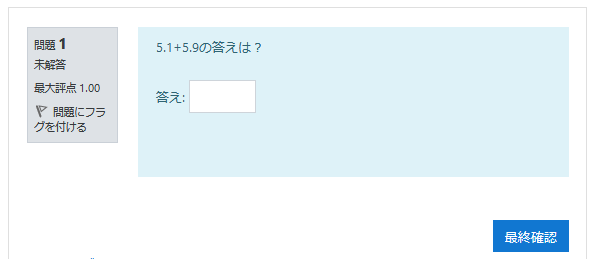If no need to generate random numbers, use “Numerical question.”

Refer to:

1. Choose [Calculated] and click [Add].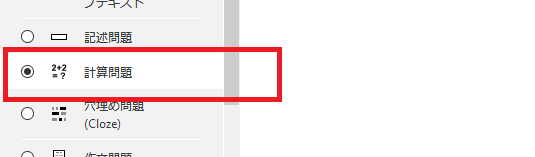2. Select “Category.” A list of all shared wildcards in this category will be displayed.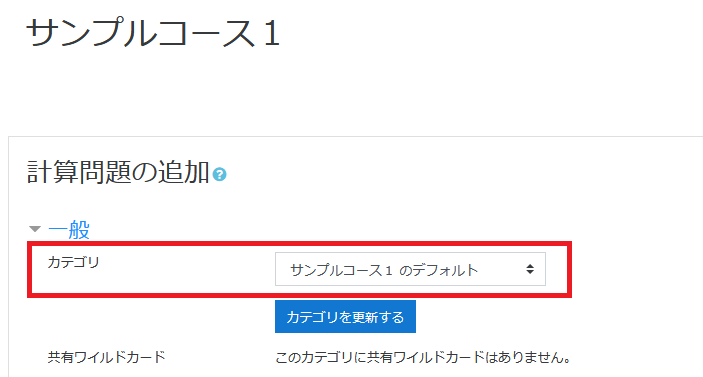3. Enter “Question name” and “Question text.” Enter “Default mark.”
“Question text” must contain all the information for students to calculate and answer. Therefore, it must contain a single wildcard enclosed in curly brackets.
In the example, since we are trying to find the answer for the area of the triangle, the question text is: ({x} and {y} are random variables).
“Find the area of the triangle with base = {x} and height = {y}.”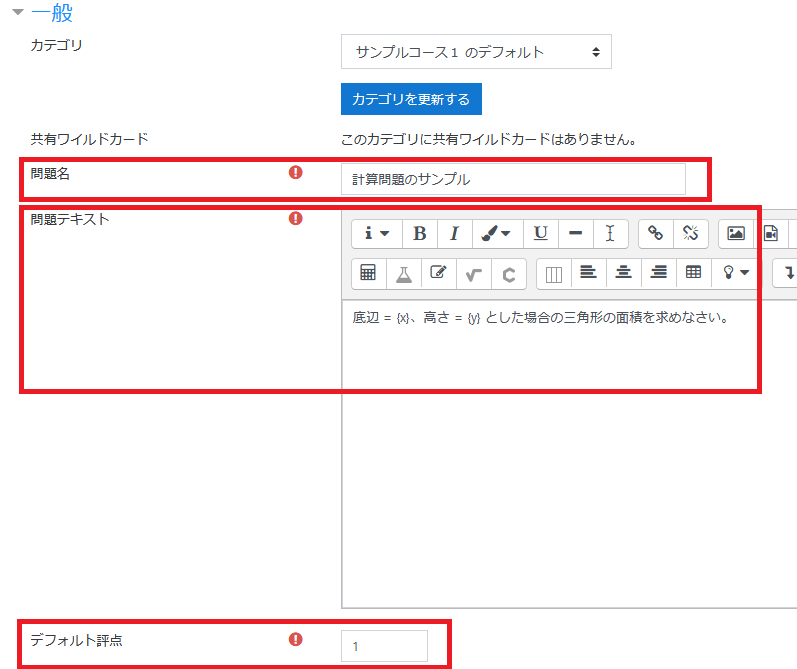4. In “Answer X formula,” enter the correct formula.
Refer to:

For “Grade,” select the grade to be given to students when answering this question. This grade is a percentage of the total points available. For example, you can give 100% to correct answer and 50% to almost correct. One of the answers must be 100%.
In “Tolerance,” specify the error tolerance. The tolerance and the tolerance type, both settings can determine the range of grades to accept. For example, if tolerance = t, correct = x, and the difference between user and correct = dx, the tolerance type is :
・Nominal scale – if dx <= t, it is considered to be correct.
・Relative frequency – if dx / x <= t, it is considered to be correct.
・Geometric mean – dx² / x² <= t², the answer is considered to be correct.
In “Answer display” and “Format” will determine the accuracy of the answer. Use these settings to select decimal point and significant digits.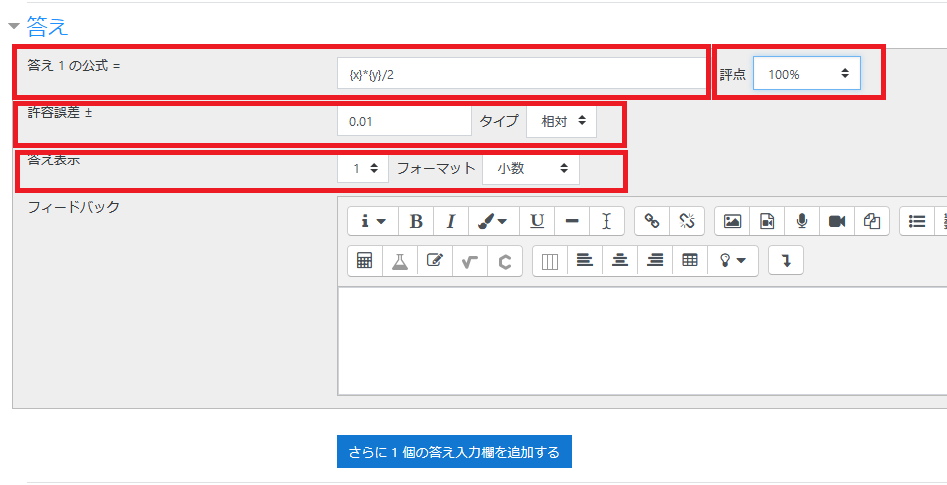5. Also you can specify “Unit.”

Refer to:
Moodle Docs“Calculated question type”
6. Click [Save changes].
7. Wildcard
For the wildcards specified in the answer formula, you need to create a series of values. These values are called “Dataset.”
Dataset used by each wildcard is as follows:
・Private Example) used only in this quiz
・Shared Example) shared with calculated questions in the same categoryClick [Next page] after specified.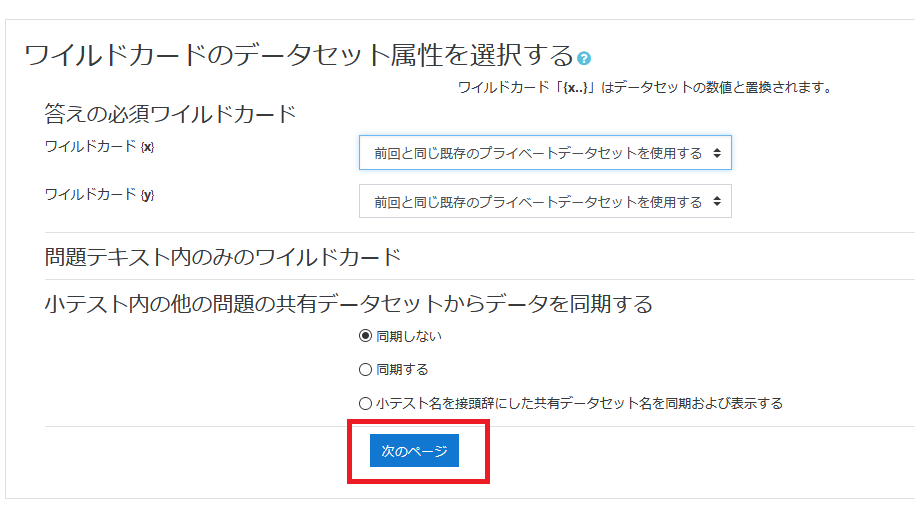8. Set the range of wild card value.
In this example, it is used with following range: <= {x} <= 10、5 <= {y} <= 15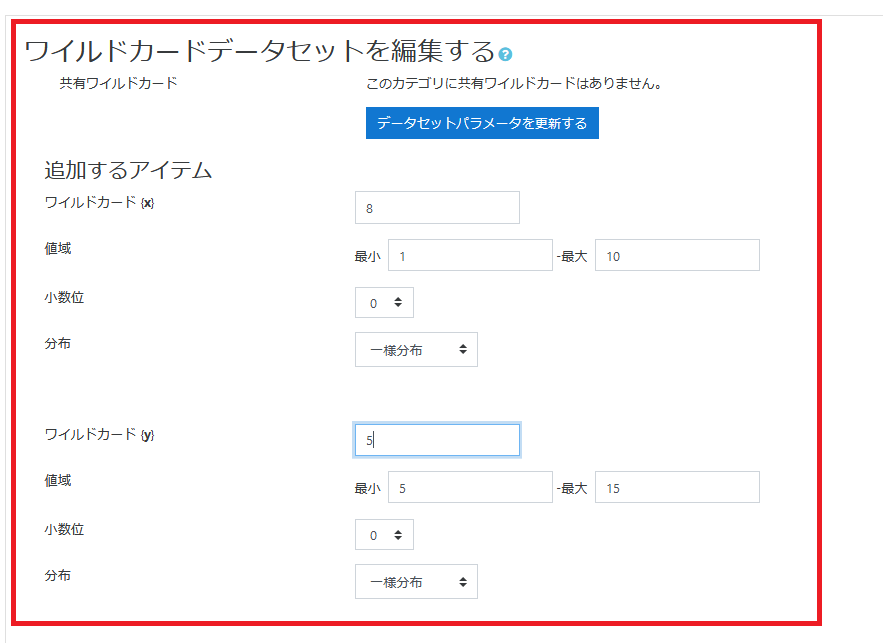9. After completing settings of wildcards value, click “Add item.”
A set of wildcards will be displayed at the bottom of the page. If there is no problem, click [Save changes].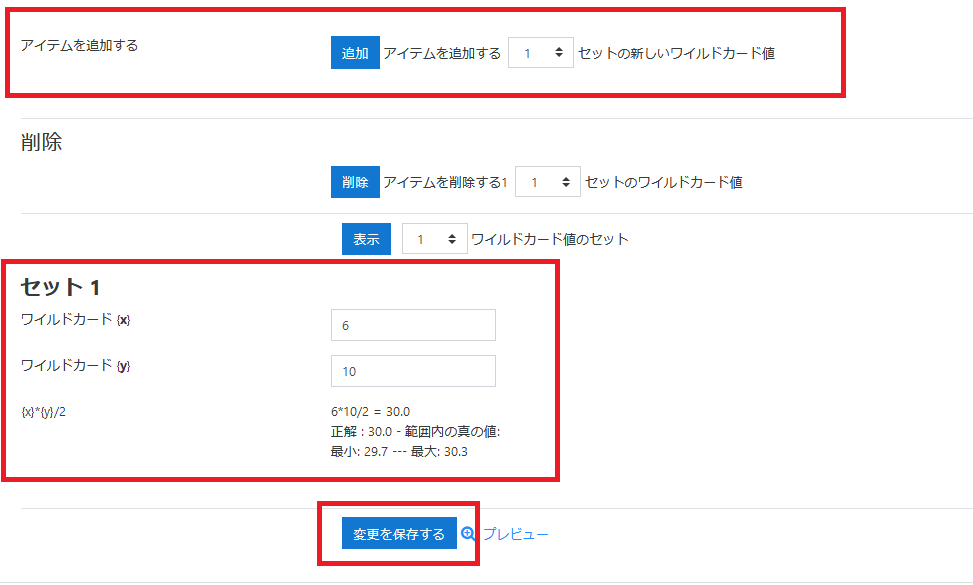10. The question will be added.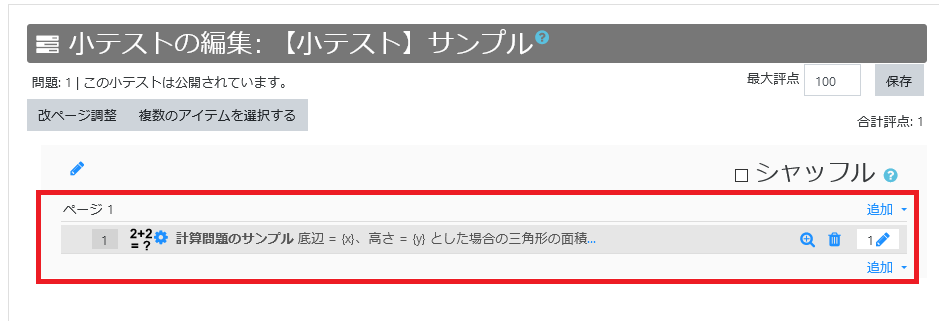11. Check the question on the preview display.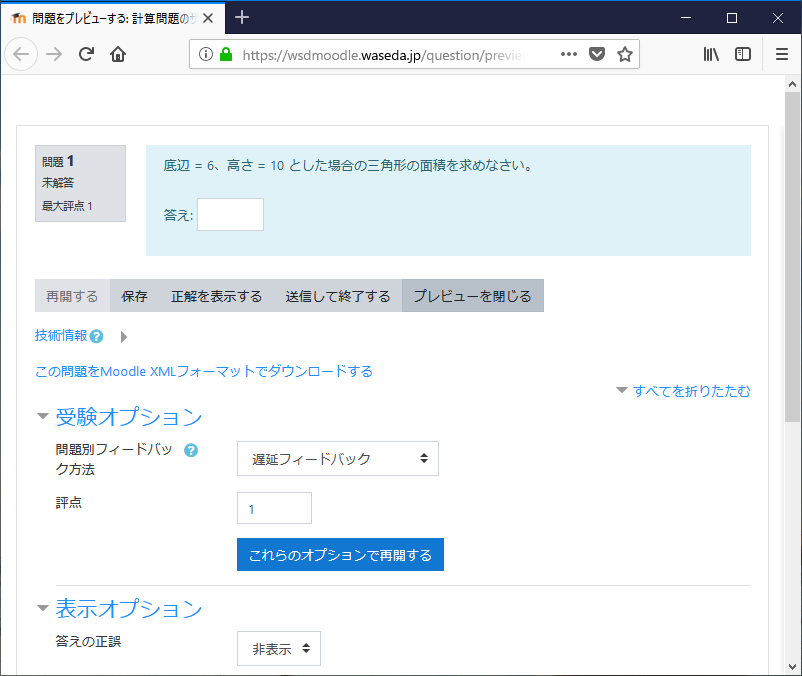Last Updated on 2021-03-30